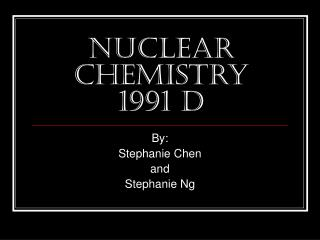DownloadDownload PresentationNuclear Chemistry 1991 D

# Nuclear Chemistry 1991 D

Télécharger la présentation## Nuclear Chemistry 1991 D

- - - - - - - - - - - - - - - - - - - - - - - - - - - E N D - - - - - - - - - - - - - - - - - - - - - - - - - - -
##### Presentation Transcript

1. Nuclear Chemistry1991 D By: Stephanie Chen and Stephanie Ng

2. 1991 D Explain each of the following in terms of nuclear models.

3. 4He 1991 D (a) The mass of an atom of the is less than the sum of the masses of two protons, two neutrons, and two electrons.

4. 4He 1991 D (a) ANSWER The mass of an atom of is less than the sum of the masses of two protons, two neutrons, and two electrons because of the “Binding Energy.” Binding energy is the energy which is used to hold the nucleus and atom together. This is the mass defect.

5. 1991 D (b) Alpha radiation penetrates a much shorter distance into a piece of material than does beta radiation of the same energy.

6. 4He 4He 2 2 1991 D (b) ANSWER Alpha radiation penetrates a much shorter distance into a piece of material that does beta radiation of the same energy because of the size. Since beta radiation is using the electron, its size of being 1/2000 of an atom is very tiny. Yet, since a atom is much larger than an electron, it is more difficult for alpha radiation ( ) to go farther.

7. 1991 D (c) Products from a nuclear fission of a uranium atom such as 90Sr and 137Ce are highly radioactive and decay by emission of beta particles.

8. 90Se 137Ce 90Se 137Ce 1991 D (c) ANSWER Since radioactivity is based on the belt of stability, we must calculate the ratio between the protons and neutrons. neutrons / protons = (90 – 34) / 34 = 1.647 neutrons / protons = (137 – 58) / 58 = 1.362 The ratio of protons to neutrons is much higher than the ideal 1 : 1 ratio. Hence, are highly radioactive and decay by emission of beta particles in which the neutrons into protons for stabilization.

9. 1991 D (d) Nuclear fusion requires large amounts of energy and to get started, whereas nuclear fission can occur spontaneously, although both processes release energy.

10. 1991 D (d) ANSWER Nuclear fusion requires large amounts of energy because of the high level of temperature the reaction must undergo in order for it to work. Yet nuclear fission can occur spontaneously despite the fact that both processes release energy, because it is considered a “chain reaction”. Fission begins whenever a neutron is bombarded into an atom, releasing more neutrons and atoms, continuously hitting each other and maintaining the process of fission.